# What is Types of Syntax Directed Translation Schemes?

There are two types of Syntax directed translation schemes which are as follows −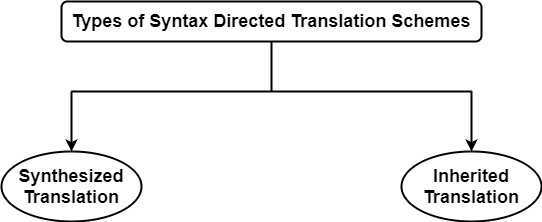Synthesized Translation

In this translation, values of variables on L.H.S of a production rule depend on the value of the variable on R.H.S of production rule.

For Example,

E → E(1) + E(2)     {E. VAL = E(1). VAL + E(2). VAL}

Here, E on L.H.S of production rule can be computed by the sum of values of E(1) and E(2) which are on R.H.S of a production rule, i.e., L.H.S variable is dependent on R.H.S variables.

In synthesized translation, the value of the synthesized attribute at the node is evaluated from the values of attributes at the children of that node in the Parse Tree.

For Example, In Productions.

E → E(1) + E(2)

E → digit

where digit = 0 | 1 | 2 |… . . | 9

The Parse Tree for string 2 + 3 will beThe complete parse tree, i.e., Parse Tree with translation will consist of a tree with each node labeled with attribute VAL.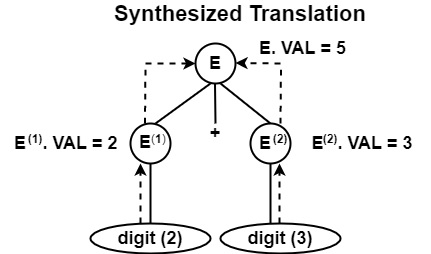Here, E. VAL represents the value of non-terminal E, computed from the values at the children of that node in Parse Tree.

Inherited Translation

In this translation, the translation of the Non-terminal on the right side of production depends on the translation of the Non-terminal on the left side of production rules.

For example, consider a production with semantic action.

A → BC                             {B. VAL = 5 ∗ A. VAL}

In this production, it can view the value of B is dependent on the value of A. So, nonterminal translation at R.H.S of production (B.VAL) depends on non-terminal translation (A.VAL) at L.H.S of production.

The value of inherited attributes is evaluated from the value of attributes at sibling and parent of that node.

For example, a complete parse tree will be

A → BC                            {B. VAL = 5 ∗ A. VAL}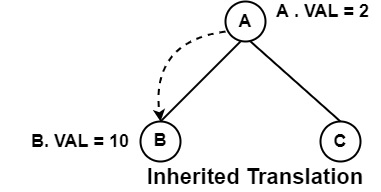If A.VAL = 2, so, B.VAL = 5 * 2 = 10

So, the value of B is computed from the parent of that node.

Example of Inherited Translation− Consider the production

A → BC {C. VAL = 2 ∗ B. VAL}

Its complete parse tree will be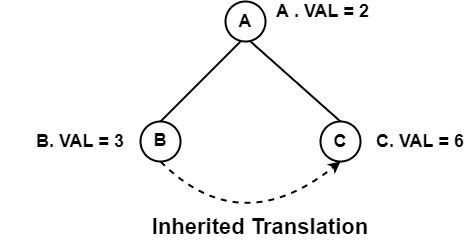Here, value at node C on its sibling B. So, in inherited translation, the value of inherited attributes at a node depends upon its parent node or Sibling node.

Translation on Parse Tree

Consider the Grammar

E → E + T

E → T

T → T ∗ F

T → F

F → digit

Following is the syntax-directed definition for the above Grammar.

ProductionSemantic Action
E → E + TE. VAL = E1. VAL + T1. VAL
E → TE. VAL = T. VAL
T → T ∗ FT. VAL = T. VAL ∗ F. VAL
T → FT. VAL = F. VAL
F → digitF. VAL = digit

Following diagram shows for Parse Tree & Complete Parse Tree for string 5 + 6 + 7.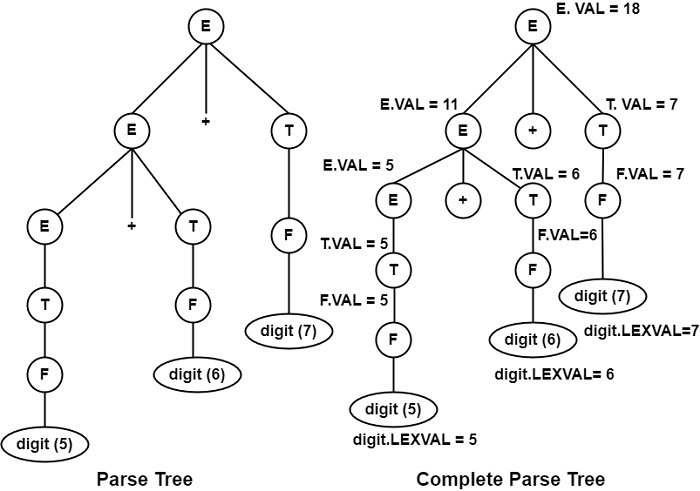Complete Parse Tree (Annotated Parse Tree) − A Parse Tree displaying the value of attributes at every node.

LEXVAL represents the values of the digits at the leaf node.